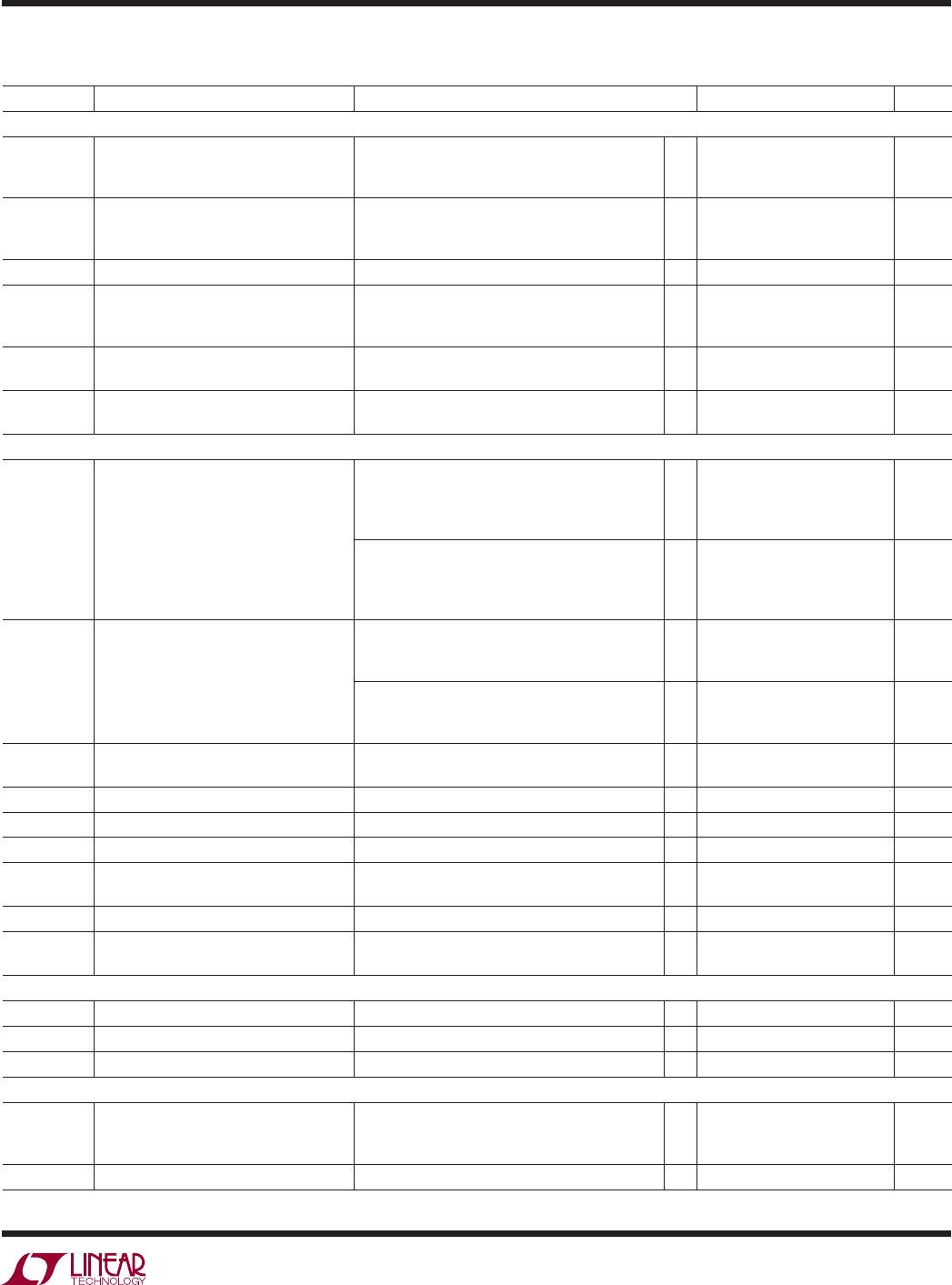# DatasheetLTC2854/LTC2855
3
285455fb
The denotes the speciﬁ cations which apply over the full operating
temperature range, otherwise speciﬁ cations are at T
A
= 25°C, V
CC
= 3.3V unless otherwise noted (Note 2).
SYMBOL PARAMETER CONDITIONS MIN TYP MAX UNITS
Driver
|V
OD
| Differential Driver Output Voltage R = ∞, V
CC
= 3V (Figure 1)
R = 27Ω, V
CC
= 3V (Figure 1)
R = 50Ω, V
CC
= 3.13V (Figure 1)
1.5
2
V
CC
V
CC
V
CC
V
V
V
Δ|V
OD
| Change in Magnitude of Driver
Differential Output Voltage for
Complementary Output States
R = 27Ω or R = 50Ω (Figure 1)
0.2 V
V
OC
Driver Common Mode Output Voltage R = 27Ω or R = 50Ω (Figure 1)
3V
Δ|V
OC
| Change in Magnitude of Driver
Common Mode Output Voltage for
Complementary Output States
R = 27Ω or R = 50Ω (Figure 1)
0.2 V
I
OZD
Driver Three-State (High Impedance)
Output Current on Y and Z
DE = OV, (Y or Z) = –7V, 12V (LTC2855)
±10 μA
I
OSD
Maximum Driver Short-Circuit Current –7V ≤ (Y or Z) ≤ 12V (Figure 2)
–250
180 ±250
300
mA
mA
I
IN
Receiver Input Current (A, B) DE = TE = 0V, V
CC
= 0V or 3.3V, V
IN
= 12V
DE = TE = 0V, V
CC
= 0V or 3.3V, V
IN
= –7V,
–100
125 μA
μA
DE = TE = 0V, V
CC
= 0V or 3.3V, V
IN
= 12V
DE = TE = 0V, V
CC
= 0V or 3.3V, V
IN
= –7V,
–145
250 μA
μA
R
IN
Receiver Input Resistance RE = V
CC
or 0V, DE = TE = 0V,
V
IN
= –7V, –3V, 3V, 7V, 12V (Figure 3)
96 125
RE = V
CC
or 0V, DE = TE = 0V,
V
IN
= –7V, –3V, 3V, 7V, 12V (Figure 3)
48 125
V
TH
Voltage
–7V ≤ B ≤ 12V
±0.2 V
ΔV
TH
= 0V
25 mV
V
OH
Receiver Output HIGH Voltage I(RO) = –4mA, A-B = 200mV, V
CC
= 3V
2.4 V
V
OL
Receiver Output LOW Voltage I(RO) = 4mA, A-B = –200mV, V
CC
= 3V
0.4 V
I
OZR
Output Current on RO
RE = V
CC
, 0V ≤ RO ≤ V
CC
±1 μA
I
OSR
Receiver Short-Circuit Current 0V ≤ RO ≤ V
CC
±85 mA
R
TERM
Receiver Input Terminating Resistor TE = V
CC
, V
AB
= 2V, V
B
= –7V, 0V, 10V
(Figure 8)
108 120 156 Ω
Logic
V
IH
Logic Input High Voltage V
CC
= 3.6V
2V
V
IL
Logic Input Low Voltage V
CC
= 3V
0.8 V
I
INL
Logic Input Current
0 ±10 μA
Supplies
I
CCS
Supply Current in Shutdown Mode DE = 0V, RE = V
CC
, TE = 0V